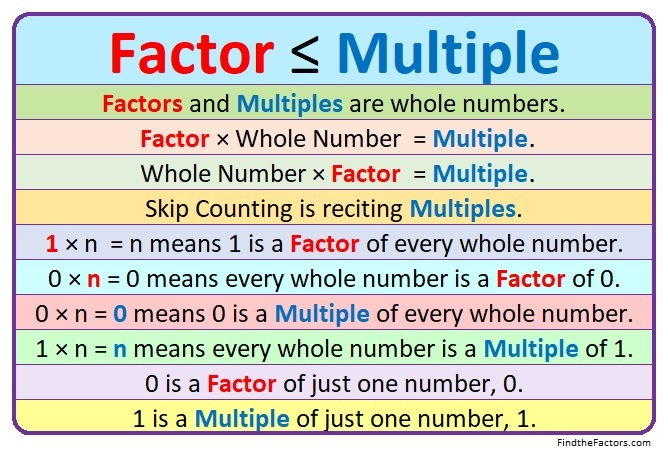# 1638 Factors and Multiples

Contents

### Mathematical Musings:

I like the way this tweet shows familiar relationships of several unfamiliar math terms.

Recalling that MANY people confuse factors with multiples, I was inspired to make something similar that will hopefully help people to know which is which:### Factors of 1638:

1638 is even, so it is divisible by 2.
1
+ 8 = 9 and 6 + 3 = 9, so 1638 is divisible by 9.

• 1638 is a composite number.
• Prime factorization: 1638 = 2 × 3 × 3 × 7 × 13, which can be written 1638 = 2 × 3² × 7 × 13.
• 1638 has at least one exponent greater than 1 in its prime factorization so √1638 can be simplified. Taking the factor pair from the factor pair table below with the largest square number factor, we get √1638 = (√9)(√182) = 3√182.
• The exponents in the prime factorization are 1, 2, 1, and 1. Adding one to each exponent and multiplying we get (1 + 1)(2 + 1)(1 + 1)(1 + 1) = 2 × 3 × 2 × 2 = 24. Therefore 1638 has exactly 24 factors.
• The factors of 1638 are outlined with their factor pair partners in the graphic below.### More about the Number 1638 and Today’s Puzzle:

1638₁₀ = 666₁₆ because 6(16² + 16¹ + 16º) = 6(256 + 16 + 1) = 6(273) = 1638.

1638 is the hypotenuse of a Pythagorean triple:
630-1512-1638.Solution:
It may be a little confusing because of the words greatest and least, but remember factor ≤ multiple so
Greatest Common Factor < Least Common Multiple.
Or simply, GCF < LCM.

Since these numbers have several digits, skip counting to find common multiples isn’t practical. An easy way to solve the puzzle is to pay attention to the exponents in the prime factorizations:
630 = 2 × 3² × 5 × 7,
1512 = 2³ × 3³ × 7, and
1638 = 2 × 3² × 7 × 13.

Rewrite the prime factorizations to contain all the bases used in any of the prime factorizations with the appropriate exponents:
630 = 2¹ × 3² × 5¹ × 7¹ × 13º,
1512 = 2³ × 3³ × 5º × 7¹ × 13º, and
1638 = 2¹ × 3² × 5º × 7¹ × 13¹.

Write the bases using the SMALLEST exponents for the Greatest Common Factor:
GCF = 2¹ × 3² × 5º × 7¹ × 13º = 2 × 3² × 7 = 126.

Write the bases using the LARGEST exponents for Least Common Multiple:
LCM = 2³ × 3³ × 5¹ × 7¹ × 13¹ = 98280.
(Aren’t you glad we didn’t skip count to find it!)

Is GCF < LCM?
126 < 98280. Most certainly!

As you might expect, 630-1512-1638 is 126 times (5-12-13).

This site uses Akismet to reduce spam. Learn how your comment data is processed.